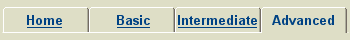Classpad Help Series - AdvancedClick a title to play a short movie in the right hand pane.Choose PDF to open a printable help sheet in a new window.Starting Out In Main 070 Define CIS PDF 071 Define Function With Many Variables PDF 073 Complex Number Basics PDF 074 Polar To Rectangular Coordinate Conversions PDF 080 Normal Probability Calculations PDF 081 Inverse Normal Probability Calculations PDF 082 Random Sample From Normal Distribution PDF 083 Binomial Distribution Calculations PDF 085 Random Sample From Binomial Distribution PDFWorking In Main 170 Volume Of Revolution PDF 171 Implicit Differentiation PDF 172 Inverse Of A Function PDF 180 Vector Basics PDF 181 Vector Tips PDFSolving Equations 270 Use Of dSolve In Growth And Decay Problems PDF 271 Use dSolve With Simple Harmonic Equations PDFGraph and Table 370 Polar Graphing PDF 371 Parametric Graphing PDF 372 Table Of Binomial Probabilities PDFStatistics and Lists 470 Normal Probability Calculations PDF 471 Inverse Normal Probability Calculations PDF 472 Confidence Interval From List Data PDF 473 Confidence Interval From Summary Data PDF 474 Binomial Distribution Calculations PDF 475 Graph Binomial Distribution PDFeActivities 670 Trapped Areas PDF 671 Differentiation From First Principles PDF 672 Average Rate Of Change Of Function PDF 675 Normal Probabilities Using Solve PDF 676 Vectors Closest Approach 1 PDF 677 Vectors Closest Approach 2 PDF 680 Geometry Link PDFGeometry 770 Apply Matrix Transformation PDF 771 Find Transformation Matrix PDF 780 Animation Basics PDF 781 Angle in a Semi-Circle 2 PDF 784 Cyclic Quadrilaterals 2 PDFSequence 880 Explicit From Recursive PDFOther Applications 980 Enable And Set Shift Keys PDF 981 Ending Screens PDF 982 Create An Ending Screen PDF 983 Use Library For Global Access PDF 984 Example Small Programs PDF 985 Create A Small Program PDFComplete Collection The complete collection of help sheets can be downloaded as a single pdf document CP000 Classpad Help Series.pdf.